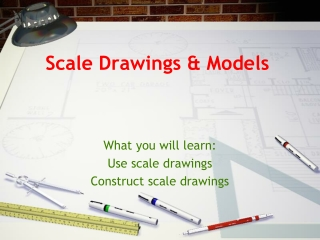DownloadDownload PresentationScale Drawings & Models

# Scale Drawings & Models

Télécharger la présentation## Scale Drawings & Models

- - - - - - - - - - - - - - - - - - - - - - - - - - - E N D - - - - - - - - - - - - - - - - - - - - - - - - - - -
##### Presentation Transcript

1. Scale Drawings & Models What you will learn: Use scale drawings Construct scale drawings

2. Vocabulary • Scale drawing/scale model: represent an object that is too large or too small to be drawn

3. Scale Scale: relationship between the measurements on the drawing and the real object Example: 1 inch = 3 feet (One inch represents an actual distance of 3 feet)

4. Scale Factor Scale factor: the ratio of a length on a scale drawing to the length on the real object Example: If a scale model has a scale of 2 inches = 16 inches. The scale factor is 2 or 1 16 8 The lengths and widths of objects of a scale drawing or model are proportional to the lengths and widths of the actual object.

5. Example 1: Find Actual Measurements A set of landscape plans shows a flower bed that is 6.5 inches wide. The scale on the plans is 1 inch = 4 feet. What is the width of the actual flower bed?

6. Example 3: Construct a Scale Drawing A garden is 8 feet wide by 16 feet long. Make a scale drawing of the garden that has a scale of 1 in. = 2ft. 4 Step 1: Find the measure of the garden’s length on the drawing. Let x represent the length. On the drawing, the length is 2 inches

7. Step 2: Find the measure of the garden’s width on the drawing. Let w represent the width.

8. YourTurn! On a set of architectural drawings for an office building, the scale is 1/2” = 3 feet. Find the actual length of each room. Lobby: 2 inches Cafeteria: 8.25 inches

9. YourTurn,Again! In an illustration of a honey bee, the length of the bee is 4.8 cm. The actual size of the honeybee is 1.2 cm. What is the scale of the drawing? 4.8 cm = 1cm 1.2 cm x cm 4.8x = 1.2 x = .25 The scale of the drawing is 1 cm = .25cm